×#### Thank you for registering.

One of our academic counsellors will contact you within 1 working day.

Click to Chat

1800-1023-196

+91-120-4616500

CART 0

• 0

MY CART (5)

Use Coupon: CART20 and get 20% off on all online Study Material

ITEM
DETAILS
MRP
DISCOUNT
FINAL PRICE
Total Price: Rs.

There are no items in this cart.
Continue Shopping• Complete JEE Main/Advanced Course and Test Series
• OFFERED PRICE: Rs. 15,900
• View Details

Revision Notes on Electromagnetic Induction

Magnetic flux:-

Magnetic flux lined with the surface is defined as the product of area and component of B perpendicular that area.

and

ϕB = µnAH

Here, µ is the permeability of the medium, n is the number of turns, A is the area and H is the magnetic field intensity.

(a) When θ = 90º, cosθ = 0. So, ϕB = 0

This signifies, no magnetic flux is linked with surface when the field is parallel to the surface.

(b) When θ = 0º, cosθ = 1. So,(ϕB)max = 1

This signifies, magnetic flux linked with a surface is maximum when area is held perpendicular to the direction of field.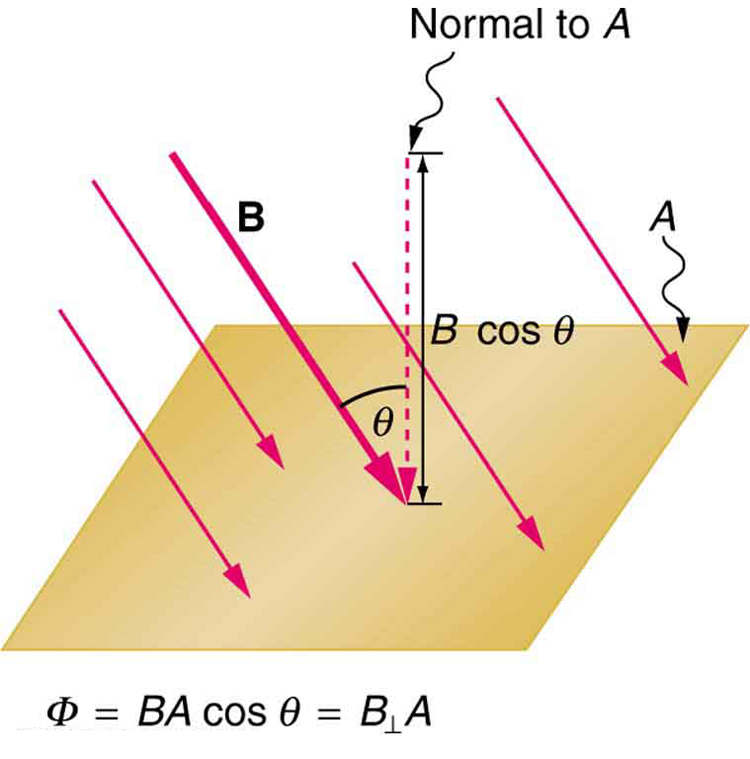(a) Whenever magnetic flux linked with a circuit changes, an e.m.f is induced in it.

(b) The induced e.m.f exists in the circuit so long as the change in magntic flux linked with it continues.

(c) The induced e.m.f is directly proportional to the negative rate of change of magnetic flux linked with the circuit.

So, E = -dϕB/dt

Negative sign is due to the direction of induced e.m.f.

Induced electric field:-

Lenz’s Law:-

It states that direction of induced e.m.f. is such that it tends to oppose the very csause which produces it.

The induced e.m.f. always tends to oppose the cause of its production.

Motion of a straight conductor in a uniform magnetic field:-

(a) W = Bevl

(b) Motional e.m.f, E = Bvl

(c) Induced current, I = E/R = Blv/R

(d) F = IlB = B2l2v/R

(e) P = Fv = IlBv = B2l2v2/R

(f) H = I2R = B2l2v2/R

Motion of a loop in a magnetic field when whole of the coil is in the magnetic field:

(a) Motional e.m.f , E = 0

(b) Resultant Current, I = 0

(c) Force, F = 0

(d) Power, P = 0

Motion of a loop in a magnetic field when a part of the loop is out of the magnetic field:-

(a) ϕB = Blx

(b) Induced e.m.f , E = Blv

Power:-

P = I2R = E2/R

P = B2l2v2/R       (Since, E = Blv)

(a) Coil out of field:- ϕB =0, E = 0, P = 0

(b) Coil entering the magnetic field:-

E = a negative constant

P = a positive constant

(c) Coil moving in the magnetic field:-

ϕB = Constant

E = 0

P = 0

(d) Coil leaving the magnetic field:-

E = a positive constant

P = a positive constant

(e) Coil out of magnetic field:-

ϕB = 0

E = 0

P = 0

Self-Induction:- Self Induction of a circuit is defined as the property of the circuit by virtue of which it tends to oppose a change in the strength of current, through it, by inducing an e.m.f. in itself.

(a) Magnetic flux, ϕB = LI

Here L is the coefficient of self -induction.

(b) e.m.f., E = -L [dI/dt]

(c) L = µ0 µrnNA

Here, n is the number of turns per unit length

Series and parallel combination:-

(a) L = L1+L2 (If inductors are kept far apart and joined in series)

(b) L = L1+L2±2M   (If inductors are connected in series and they have mutual inductance M)

(c) 1/L = 1/L1 + 1/L2 (If two conductors are connected in parallel and are kept for apart)

(d) M = K√L1L2      (If two coils of self-inductances, L1 and L2 are over each other)

Inductance of wire:-

L = µ0l/8π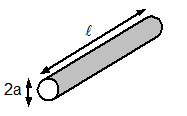Inductance of hollow cylinder:-

L = µ0l/2π [ln 2l/a -1],  l >> a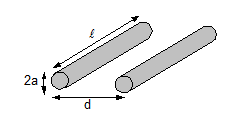Inductance of parallel wires:-

L = µ0l/π [ln d/a -1], l >> d, d >> aInductance of Coaxial conductor:-

L = µ0l/π [ ln b/a]

Inductance of Circular loop:-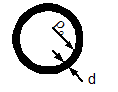L = µ0l/2π [ln 4l/d – 2.45]

l = -2πρ0, ρ0 >> d

Inductance of Solenoid:-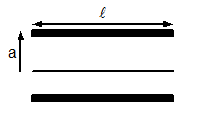L = µ0N2S/l

L >> a

Inductance of Torus (of circular cross section):-

L = µ0N2 [ρ0 - √ ρ02 – a2]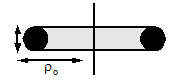Inductance of Sheet:-L = µ02l [ln (2l/b+t) + 0.5]

Energy stored in an conductor:-

(a) W = ½ LI2

Here L is the coefficient of self -induction.

(b) UB = B2/2µ0

Mutual Induction:-

Mutual induction of two circuits is the phenomenon where a current changing in the first coil results in the induction of an e.m.f. in the second.

Coefficient of Mutual Induction:-

ϕB = MI and E = -M[dI/dt]

Here M is called the coefficient of mutual induction of two circuits.

The value of M, M =  µ0 µrn1 N2 A

M depends upon,

(a) Area of cross-section of the two coils

(b) Number of turn of each coil

(c) Distance between the two coils

(d) Nature of material used as core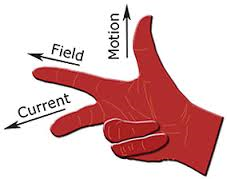Fleming’s right hand rule:-

Stretch first finger, central finger and the thumb of your right hand in three mutually perpendicular directions. If the first finger points towards the magnetic field, thumb points towards the direction of motion of conductor, the direction of central finger gives the direction of induced current set up in the conductor.

Coil rotating in a uniform magnetic field:-

(a) Magnetic flux, ϕB = µnaH [cos ωt]

(b) Electromagnetic Induction, E = µnaωH [sin ωt]

(c) Current, I = [µnaωH[sin ωt]]/R

Growth and decay of current in LR circuit:-

(a) I = I0(1-e-t/τ)         (for growth), Here τ = L/R

(b) I = I0e-t/τ               (for decay), Here τ = L/R### Course Features

• 728 Video Lectures
• Revision Notes
• Previous Year Papers
• Mind Map
• Study Planner
• NCERT Solutions
• Discussion Forum
• Test paper with Video Solution